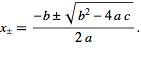# Solve 6x^2+3x-18=0 Using The Quadratic Formula?

The quadratic formula tells you the solution to
ax^2 + bx + c = 0
is given by
x = (-b ± √(b^2 - 4ac))/(2a)

You have an equation in which a=6, b=3, c=-18, so the formula gives
x = (-3 ± √(3^2 - 4(6)(-18))/(2*6)
x = (-3 ± √(9+432))/12
x = (-3 ± √441)/12
x = (-3 ± 21)/12
x = {-24/12, 18/12}
x = {-2, 3/2}
thanked the writer.
This is simple:

6x2 + 3x - 18 = 0
Dividing the equation by 3, we get:
2x2 + x - 6 = 0
2x2 + 4x - 3x - 6 = 0
2x(x + 2) -3(x + 2)
(2x-3)(x+2)=0
x= 3/2
x=-2
thanked the writer.
Shelia, first let's see what the quadratic formula is, ok?

x = [-b ±b2-4ac]/2a

6x2 + 3x -18 = 0

The values from the quadratic formula that we extract from this equation are:

a = 6
b = 3
c = -18

Now, all you have to do is put these values in the quadratic formula and you will get your answer
x = [-b ±b2-4ac]/2a

x =  [-3 ±32-4.6.-18]/2.6
(I am using a period to show a multiplication sign so that you do not confuse it with 'x' in the equation)

x = [-3 ± √9 + 432]/12
x = [-3
± √441]/12
x = [-3
± 21]/12

Now, the '
±' sign shows that the value can be either positive or negative.

So, we will solve for each. Now our two new equations are:

x = [-3 + 21]/12
x = 1.5

and

x = [-3 - 21]/12
x = -2

So, the two values for x are 1.5 and -2.

About how we type all the mathematical symbols, I use MS-Word for that. You can also use MS-Excel if you want to. Go to 'Insert' and choose 'Symbols'. Here, you would only be given a few options but an option would say, 'More symbols'. Choose that and you would probably find the symbol you are looking for.
I use MS-Office 2007. This also has inbuilt equations that you can choose from Insert 'Equations'. I think previous issues of Word would also have them. You just need to browse around a little. Use 'x' or '*' to show multiplication. You can square a number/ alphabet by writing it, like x2. Then select 2 and click on x2 on the tool bar to make it a superscript.
I think it would all be very clear to you now. Have fun!

thanked the writer.
Since the question mentions the quadratic formula, the problem should probably read 6x2 + 3x – 18 = 0. The quadratic formula is shown in the picture. Following the formula, X= (-3+(SQRT(9-4*6*(-18))))/12 and x = (-3-(SQRT(9-4*6*(-18))))/12. Or, doing the arithmetic, x=1.5 and x=-2.thanked the writer.
Here is the solution:
6x2 + 3x - 18 = 0
6x2 +12x - 9x - 18= 0
6x(x+2) -9(x+2) = 0
(6x -9)(x+2)= 0
x = -2 is one answer
x= 9/6 or 2/3 is second answer
thanked the writer.
Anonymous commented
why didn't you divide the original equation by 3 at first? that's why you're second solution is wrong.